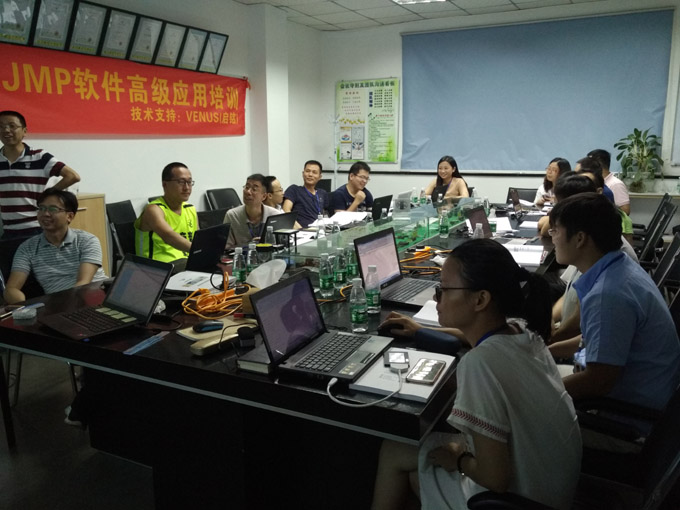CoursesContact： Phenix Zhu Tel： +86-21-33729081 Email： info@venusquality.com

Current location：Home > Courses > Advanced JMP Course

Description:

This course covers JMP software's approach to data manipulation and analysis, measurement systems analysis, design of experiments, and statistical process control in direct support of DMAIC breakthrough projects.

Duration:

Two(2)days

Benefits:

Learn how to

import and manipulate data

perform data analysis for a Six Sigma projects

design and analyze an experiment

create control charts.

Who Should Attend:

Six Sigma professionals, Six Sigma team members

Key Topics:

Basic JMP

Type of JMP Windows

Work Sheet

Generate Normal Data

Make Patterned Data (Text, data)

Graph functions

Scatter plot

Histogram

Probability plot

Probability Distribution Plot

Basic Statistic Overview

1 sample Z-test and confidence interval

1 sample t-test

2 sample t-test

Paired t-test

F-test

1 test and confidence interval of a proportion

2 test and confidence interval of two proportion

Multi-Vari Analyze

Main Effect Plot

Multi-vari chart

One way ANOVA

Basic of Hypothesis test

One way ANOVA (Stacked)

One way ANOVA (unstuck)

Graph &Compare

Two way ANOVA

Balance ANOVA

General linear fully nested ANOVA

Correlation and Regression

Correlation Analysis

Regression

Step wise regression

Best fits regression

Full factorial DOE

Basic of DOE

Design of Experiment

Center Point& Blocking

Response Surface Designs Overview

3k Factorial Designs

Response Surface Designs (Box-Behnken Designs, Central Composite Designs)

Fractional Factorial DOE

Reliability Analyze

Basic of Reliability Analysis

Estimate test plan

Accelerated life test plan

Distribution analyze (right censoringweixin：venusgroup